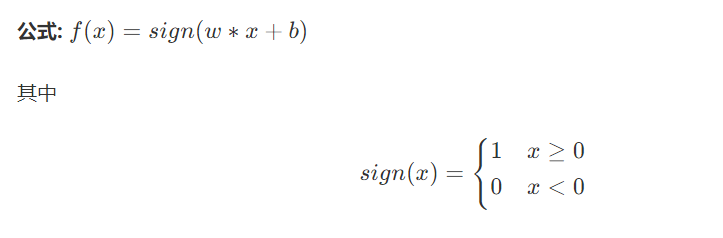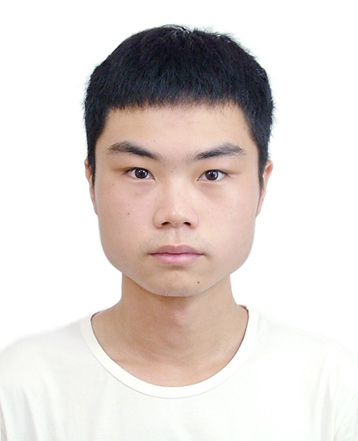# 机器学习之神经网络

+关注继续查看

## 多层神经网络## BP神经网络

BP神经网络好的地方就是我们完全不必事先考虑怎么用数学方程来描述输入和输出之间的关系，转而考虑的是设计一个N层神经网络，而要多少层、每层要多少个节点就可以我们根据经验来设计，可通过不同的网络模型来看哪个模型能更好地拟合。

BP神经网络其实很直观很好理解，整个过程如下。f2(e)节点对应的权重为w(x1)2w(x2)2，则有y2=f2(w(x1)2+w(x2)2)f3(e)节点对应的权重为w(x1)3w(x2)3，则有y3=f3(w(x1)3+w(x2)3)f5(e)对应的权重为w15w25w35，则y5=f5(w15y1+w25y2+w35y3)## 误差逆传播nodej=iwjixjiEdwji=Ednodejnodejwji=Ednodejiwjixjiwji=Ednodejxji

Edwji=Edyjyjnodejxji=(tjyj)yj(1yj)xji

wjiwjiηEdwji=wji+η(tjyj)yj(1yj)xji

δj=(tjyj)yj(1yj)
wjiwji+ηδjxji

Ednodej=kAEdnodeknodeknodej=kAEdnodeknodekf(nodej)f(nodej)nodej=kAδkwkjf(nodej)nodej=kAδkwkjf(nodej)(1f(nodej))=f(nodej)(1f(nodej))kAδkwkj

E=λ1m1mEd+(1λ)w2

1. 模拟退火
2. 随机梯度下降
3. 多个不同的初始点

## 实现3-5-1网络

import numpy as np
def nonlin(x,deriv=False):
if(deriv==True):
return x*(1-x)
return 1/(1+np.exp(-x))
X = np.array([[0,0,1],
[0,1,1],
[1,0,1],
[1,1,1]])
y = np.array([,
,
,
])
np.random.seed(1)
syn0 = 2*np.random.random((3,5)) - 1
syn1 = 2*np.random.random((5,1)) - 1
for j in range(60000):
l0 = X
l1 = nonlin(np.dot(l0,syn0))
l2 = nonlin(np.dot(l1,syn1))
l2_error = y - l2
if (j% 10000) == 0:
print("Error:" + str(np.mean(np.abs(l2_error))))
l2_delta = l2_error*nonlin(l2,deriv=True)
l1_error = l2_delta.dot(syn1.T)
l1_delta = l1_error * nonlin(l1,deriv=True)
g = 0.01
syn1 += g * l1.T.dot(l2_delta)
syn0 += g * l0.T.dot(l1_delta)
print(l2)

====广告时间，可直接跳过====

=========================9 028 01357 0573 0640 0940 01711 01025 01782 0878 0
sea-boat

276

0